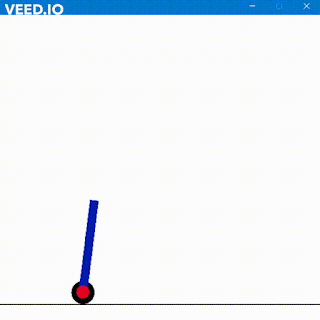## ASYNCHRONOUS COUNTER

Asynchronous means in terms of simple definition without external clock synchronization.
The output always remains free from clock signal.
Generally the first FF is clocked with main external clock and each of next FF have output of previous FF as their clock. This helps in reducing the number of FFs and additional gates hence requires less complexity.
Now coming to the special "MOD" term. It basically stands for modulus.

When you have to design a Mod-Y counter then the basic steps include
1. The equation -: 2x  = Y.
2.  Now find value of X if you know basic Maths. You can use logarithms

Thus after getting the value of X you basically get how many FFs are required hence you require X FFs to design Mod- Y UP or Down counter.

Here is the block diagram of Mod-16 or 4bit Asynchronous Counter

Now For Mod-16 we have value of X as 4 hence 4 FFs

Here is the code to test this

module dff(d,clk,q);
input d;
input clk;
wire d;
wire clk;
output q;
reg q;
initial
q = 1'b0;
always @(posedge clk)begin
q <= d;
end
endmodule
//************************Test Bench******************//
module FOURbit_up_B();
reg clk;
reg d;
wire [3:0]q;
initial
clk = 0;
always
#1 clk = !clk;
dff a1(!q,clk,q);
dff a2(!q,!q,q);
dff a3(!q,!q,q);
dff a4(!q,!q,q);
endmodule

This was Aynchronous Up counter.
Pay attention here that clock input to each FF is ~Q (Q bar). Thus for Down counter the clock input after 1st FF will be from Q and not   ~Q (Q bar)

Here is the block diagram for 4 bit Down Asynchronous Counter

Notice the clock inputs to each FF after 1st FF.

Here is the code for Down Counter 4 bit

module dff(d,clk,q);
input d;
input clk;
wire d;
wire clk;
output q;
reg q;
initial
q = 1'b0;
always @(posedge clk)begin
q <= d;
end
endmodule

module FOURbit_up_B();
reg clk;
reg d;
wire [3:0]q;
initial
clk = 0;
always
#1 clk = !clk;
dff a1(!q,clk,q);
dff a2(!q,q,q);
dff a3(!q,q,q);
dff a4(!q,q,q);
endmodule

and heres the simulation wave window from Xilinx iSim

Mail or Comment for any flaws here or if you have any doubts.

So Long

1.Hello, sir.. can you share the testbench code for the counter up.?

2.This comment has been removed by the author.

3.It is already present in the code above.
Isn't it working for you?

4.Sir it should count till 9 and then Repeat right???

1.Why till 9?
He has used 4 FFs whose maximum is 1111 i.e. 15 which is clear from the wave view.
Are u trying BCD ?

5.Red lines are coming in my output
I think sone delay problem with the codes

### Self Balancing Robot using Machine Learning

Hola Amigos, I have always loved inverted pendulums. They are very fascinating to me and I play with them a lot. In real life, I have m...